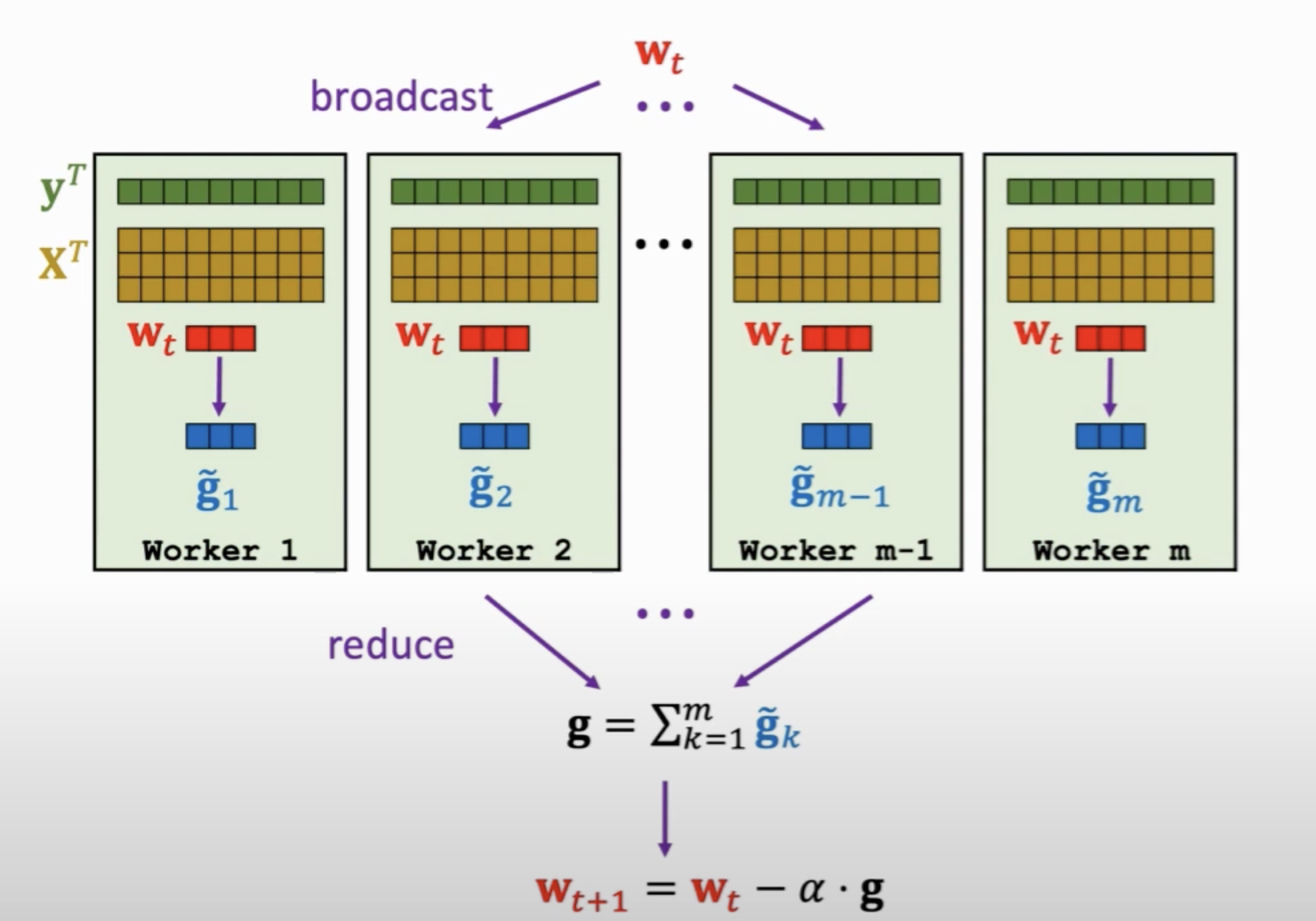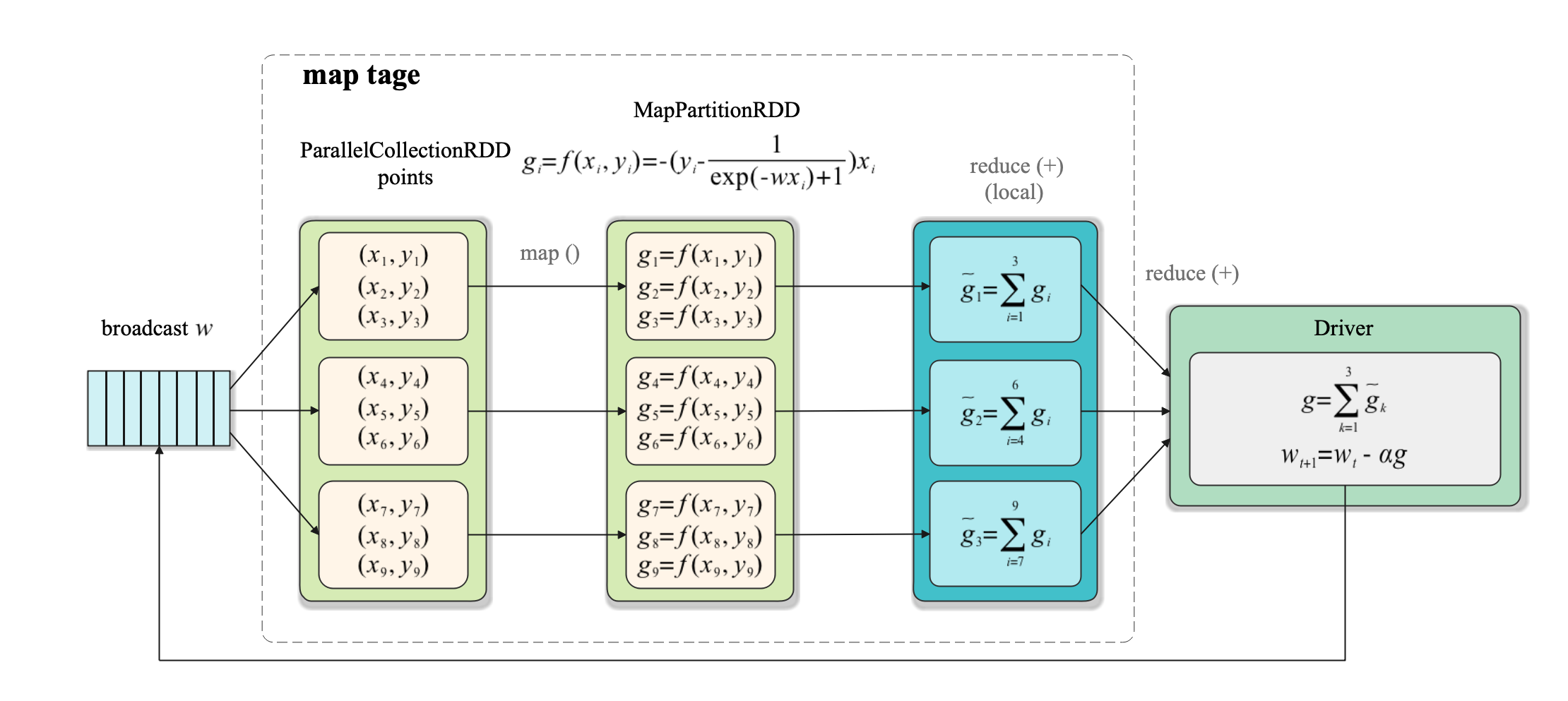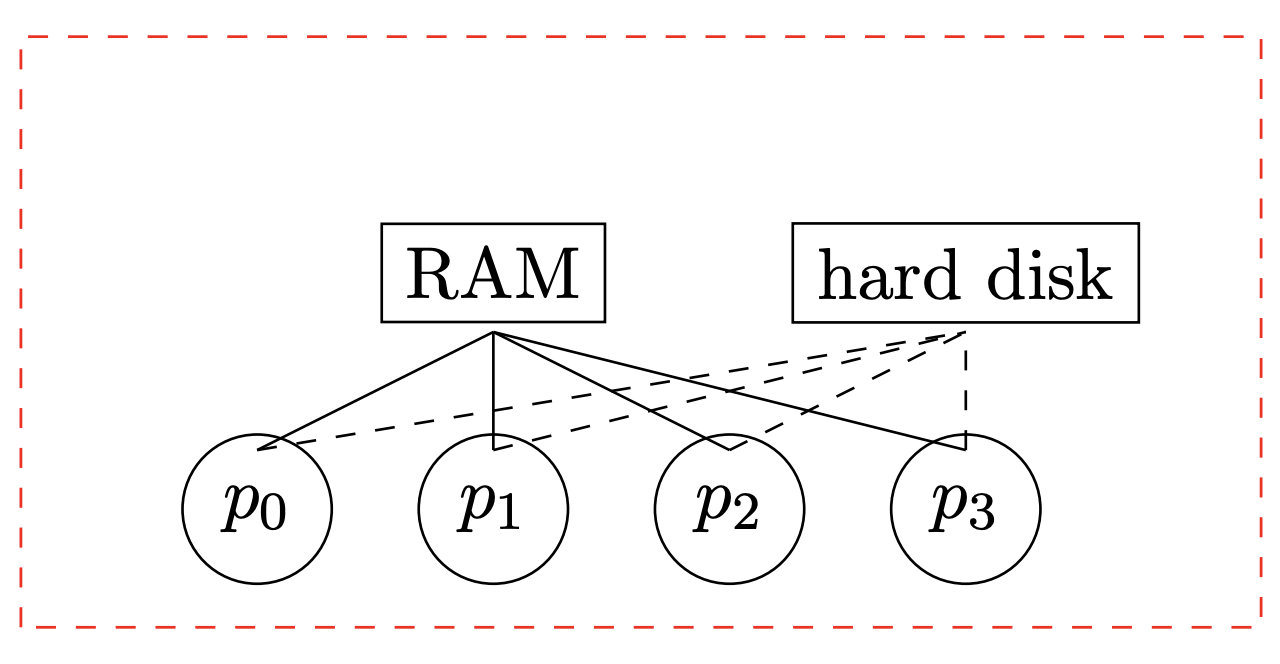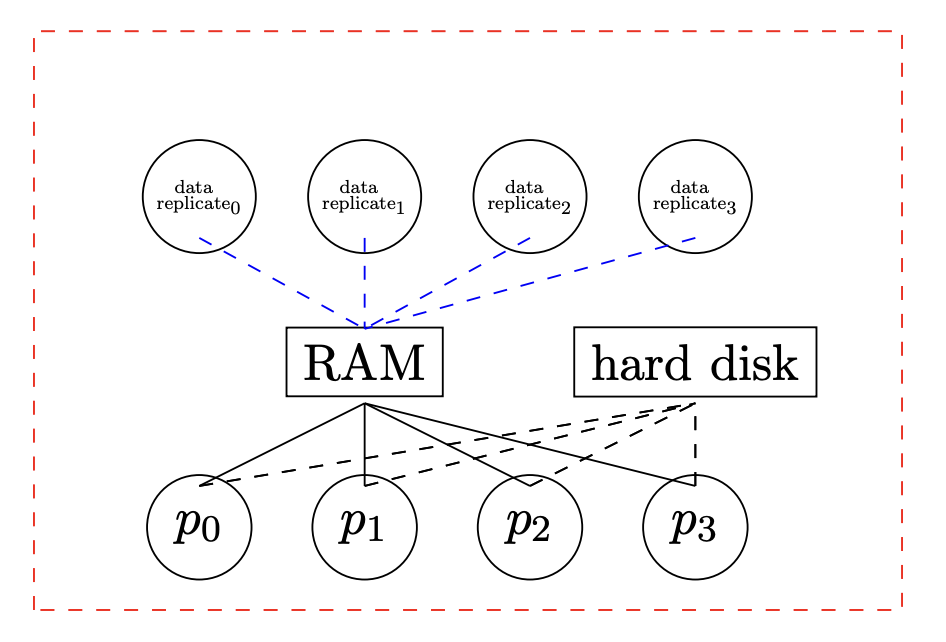<input id="ohw05"></input>
• <var id="ohw05"></var>
• <code id="ohw05"><cite id="ohw05"></cite></code>
<label id="ohw05"></label>
<var id="ohw05"></var>
• # 分布式機器學習：邏輯回歸的并行化實現（PySpark）

## 1. 梯度計算式導出

我們在博客《統計學習：邏輯回歸與交叉熵損失（Pytorch實現）》中提到，設$$w$$為權值(最后一維為偏置)，樣本總數為$$N$$$$\{(x_i, y_i)\}_{i=1}^N$$為訓練樣本集。樣本維度為$$D$$$$x_i\in \mathbb{R}^{D+1}$$（最后一維擴充），$$y_i\in\{0, 1\}$$。則邏輯回歸的損失函數為：

$\mathcal{l}(w) = \sum_{i=1}^{N}\left[y_{i} \log \pi_{w}\left(x_{i}\right)+\left(1-y_{i}\right) \log \left(1-\pi_w\left(x_{i}\right)\right)\right]$

這里

\begin{aligned} \pi_w(x) = p(y=1 \mid x; w) =\frac{1}{1+\exp \left(-w^{T} x\right)} \end{aligned}

寫成這個形式就已經可以用諸如Pytorch這類工具來進行自動求導然后采用梯度下降法求解了。不過若需要用表達式直接計算出梯度，我們還需要將損失函數繼續化簡為：

$\mathcal{l}(w) = -\sum_{i=1}^N(y_i w^T x_i - \log(1 + \exp(w^T x_i)))$

可將梯度表示如下：

$\nabla_w{\mathcal{l}(w)} = -\sum_{i=1}^N(y_i - \frac{1}{\exp(-w^Tx)+1})x_i$

## 2. 基于Spark的并行化實現

邏輯回歸的目標函數常采用梯度下降法求解，該算法的并行化可以采用如下的Map-Reduce架構：先將第$$t$$輪迭代的權重廣播到各worker，各worker計算一個局部梯度（map過程），然后再將每個節點的梯度聚合（reduce過程），最終對參數進行更新。

在Spark中每個task對應一個分區，決定了計算的并行度(分區的概念詳間我們上一篇博客Spark: 單詞計數(Word Count)的MapReduce實現(Java/Python) )。在Spark的實現過程如下：

• map階段： 各task運行map()函數對每個樣本$$(x_i, y_i)$$計算梯度$$g_i$$， 然后對每個樣本對應的梯度運行進行本地聚合，以減少后面的數據傳輸量。如第1個task執行reduce()操作得到$$\widetilde{g}_1 = \sum_{i=1}^3 g_i$$ 如下圖所示：

• reduce階段：使用reduce()將所有task的計算結果收集到Driver端進行聚合，然后進行參數更新。在上圖中，訓練數據用points:PrallelCollectionRDD來表示，參數向量用$$w$$來表示，注意參數向量不是RDD，只是一個單獨的參與運算的變量。

此外需要注意一點，雖然每個task在本地進行了局部聚合，但如果task過多且每個task本地聚合后的結果（單個gradient）過大那么統一傳遞到Driver端仍然會造成單點的網絡平均等問題。為了解決這個問題，Spark設計了性能更好的treeAggregate()操作，使用樹形聚合方法來減少網絡和計算延遲，我們在第5部分會詳細敘述。

## 3. PySpark實現代碼

PySpark的完整實現代碼如下：

from sklearn.datasets import load_breast_cancer
import numpy as np
from pyspark.sql import SparkSession
from operator import add
from sklearn.model_selection import train_test_split
from sklearn.metrics import accuracy_score

n_slices = 3  # Number of Slices
n_iterations = 300  # Number of iterations
alpha = 0.01  # iteration step_size

def logistic_f(x, w):
return 1 / (np.exp(-x.dot(w)) + 1)

def gradient(point: np.ndarray, w: np.ndarray) -> np.ndarray:
""" Compute linear regression gradient for a matrix of data points
"""
y = point[-1]    # point label
x = point[:-1]   # point coordinate
# For each point (x, y), compute gradient function, then sum these up
return - (y - logistic_f(x, w)) * x

if __name__ == "__main__":

X, y = load_breast_cancer(return_X_y=True)

D = X.shape
X_train, X_test, y_train, y_test = train_test_split(
X, y, test_size=0.3, random_state=0)
n_train, n_test = X_train.shape, X_test.shape

spark = SparkSession\
.builder\
.appName("Logistic Regression")\
.getOrCreate()

matrix = np.concatenate(
[X_train, np.ones((n_train, 1)), y_train.reshape(-1, 1)], axis=1)

points = spark.sparkContext.parallelize(matrix, n_slices).cache()

# Initialize w to a random value
w = 2 * np.random.ranf(size=D + 1) - 1
print("Initial w: " + str(w))

for t in range(n_iterations):
print("On iteration %d" % (t + 1))
w -= alpha * g

y_pred = logistic_f(np.concatenate(
[X_test, np.ones((n_test, 1))], axis=1), w)
pred_label = np.where(y_pred < 0.5, 0, 1)
acc = accuracy_score(y_test, pred_label)
print("iterations: %d, accuracy: %f" % (t, acc))

print("Final w: %s " % w)
print("Final acc: %f" % acc)

spark.stop()


注意spark.sparkContext.parallelize(matrix, n_slices)中的n_slices就是Spark中的分區數。我們在代碼中采用breast cancer數據集進行訓練和測試，該數據集是個二分類數據集。模型初始權重采用隨機初始化。

最后，我們來看一下算法的輸出結果。

初始權重如下：

Initial w: [-0.0575882   0.79680833  0.96928013  0.98983501 -0.59487909 -0.23279241
-0.34157571  0.93084048 -0.10126002  0.19124314  0.7163746  -0.49597826
-0.50197367  0.81784642  0.96319482  0.06248513 -0.46138666  0.76500396
0.30422518 -0.21588114 -0.90260279 -0.07102884 -0.98577817 -0.09454256
0.07157487  0.9879555   0.36608845 -0.9740067   0.69620032 -0.97704433
-0.30932467]


最終的模型權重與在測試集上的準確率結果如下：

Final w: [ 8.22414803e+02  1.48384087e+03  4.97062125e+03  4.47845441e+03
7.71390166e+00  1.21510016e+00 -7.67338147e+00 -2.54147183e+00
1.55496346e+01  6.52930570e+00  2.02480712e+00  1.09860082e+02
-8.82480263e+00 -2.32991671e+03  1.61742379e+00  8.57741145e-01
1.30270454e-01  1.16399854e+00  2.09101988e+00  5.30845885e-02
8.28547658e+02  1.90597805e+03  4.93391021e+03 -4.69112527e+03
1.10030574e+01  1.49957834e+00 -1.02290791e+01 -3.11020744e+00
2.37012097e+01  5.97116694e+00  1.03680530e+02]
Final acc: 0.923977


可見我們的算法收斂良好。

## 4.關于冗余存儲的反思

注意根據我們以上的代碼實現中的

map(lambda point: gradient(point, w)).reduce(add)


這一行中，我們求梯度的函數gradient會根據w將每一個訓練樣本點map到其對應梯度值。w的拷貝會被發送給每個計算節點的每個CPU。比如，假設我們有一個4個CPU的計算節點。默認當map過程發生時，所有被map過程需要的數據會被發往mapper，而此處每個CPU都有一個mapper，故如果該計算節點有4個CPU，我們實際上會發送4個w的拷貝到該節點，如下圖所示：之所以會這樣，是因為此處假定w會被修改，必須為每個CPU單獨存儲w拷貝以解決并發寫的問題。然而，當我們計算每一步的梯度時，w并未被修改，故此處不存在并發寫的問題。這導致我們浪費了存儲空間，因為本可將w存儲在各個節點的共享內存中的。

為了解決此問題，我們可以將w進行廣播，這樣它只會被發到每個計算節點一次（而不是每個CPU一次）。為了實現這個想法，我們將w定義為一個廣播變量來使用，如下面代碼所示：

# Initialize w to a random value
w = 2 * np.random.ranf(size=D + 1) - 1
print("Initial w: " + str(w))

for t in range(n_iterations):
print("On iteration %d" % (t + 1))

w -= alpha * g


當我們初始化w時，我們首先將其聲明為一個廣播變量。在每一輪梯度下降的迭代中，我們需要引用w的值。最后，我們在w被更新后重新廣播w。這樣，w在每個機器上被高效地存儲（每個機器一份，而不是多份）。

## 5.關于聚合效率的反思

正如我們前面所說，我們可以用性能更好的treeAggregate()操作，使用樹形聚合方法來減少網絡和計算延遲。
treeAggregate()函數原型如下：

RDD.treeAggregate(zeroValue, seqOp, combOp, depth=2)


其中zeroValue為聚合結果的初始值，seqOp函數用于定義單分區(partition)做聚合操作的方法，它第一個參數為聚合結果，第二個參數為分區中的數據變量。combOp定義對分區之間做聚合的方法，它第一個參數為第二個參數都為聚合結果。
depth為聚合樹的深度。

此處我們的聚合操作比較簡單，聚合結果初始值設置為0.0seqOpcombOp都設置為add算子即可：

g = points.map(lambda point: gradient(point, w_br.value))\


## 6. 復雜度和通信時間分析

### 6.1 復雜度

如我們在博客《數值優化：經典一階確定性算法及其收斂性分析》中所分析，梯度下降法在光滑強凸條件下有擁有線性收斂速率，其迭代次數復雜度為$$\mathcal{O}(\text{log}\frac{1}{\varepsilon})$$

盡管梯度的計算可以被分攤到個計算節點上，然而梯度下降的迭代是串行的。每輪迭代中，Spark會執行同步屏障(synchronization barrier)來確保在各worker開始下一輪迭代前w已被更新完畢。如果存在掉隊者(stragglers)，其它worker就會空閑(idle)等待，直到下一輪迭代。故相比梯度的計算，其迭代計算的“深度”(depth)是其計算瓶頸。

### 6.2 通信時間

$2\text{log}_2(p)(L + \frac{m}{B})$
這里$$p$$為除去driver節點的運算節點個數，$$L$$是節點之間的通信延遲。$$B$$是節點之間的通信帶寬。$$M$$是每輪通信中節點間傳輸的信息大小。